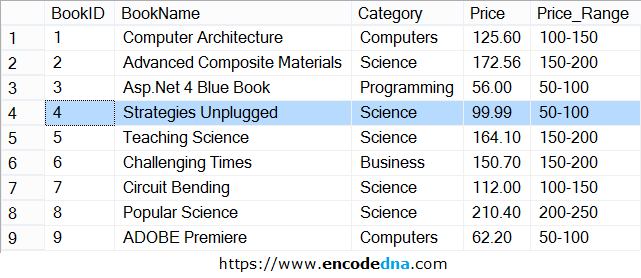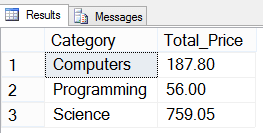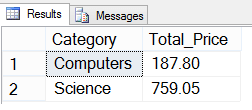﻿ Can an SQL query contain both WHERE and HAVING clause

# Can an SQL query contain both WHERE and HAVING clause

Yes, an SQL query can contain a WHERE and HAVING clause. You will use these together when you want to extract (or filter) rows for a group of data using a WHERE clause and apply a condition on the aggregate using the HAVING clause.

Here’s an example. I have a table named dbo.books and it has 5 columns and few rows of data. Here’s the dbo.books table. I’ll keep this simple.

ImageFirst, let’s see how I can use the WHERE and HAVING clause in two separate queries.

### 1)

```SELECT Category, SUM(Price) Total_Price FROM dbo.Books
WHERE Price > 150
GROUP BY Category```

In the above query (using WHERE), I’ll get total price for each category where the individual price is greater than (>) 150. Since I am using the SUM() function, I’ll have to group it using GROUP BY.

### 2)

```SELECT Category, SUM(Price) Total_Price
FROM dbo.Books
GROUP BY Category
HAVING SUM(Price) > 150```

In the second query, I am using the HAVING clause. Its on an aggregate function (SUM()). Unlike the WHERE clause, this query first returns the rows by grouping it and then filters it with the total sum using the HAVING clause.

Now, I want to do something similar (using both the above queries) in a single query. See this query first.

```SELECT Category, SUM(Price) Total_Price
FROM dbo.Books
WHERE Category IN ('Computers', 'Science', 'Programming')
GROUP BY Category```

Output (image)It returns the sum of price for individual categories. I am filtering the categories using the WHERE clause. I don’t want all the categories.

Now suppose, I want to filter it further to get the rows where sum of the price is greater (>) than 150. Not all the rows.

### Unfortunately, I cannot do this in an SQL query! 👇

```SELECT Category, SUM(Price) Total_Price
FROM dbo.Books
WHERE Category IN ('Computers', 'Science', 'Programming') AND
SUM(Price) > 150          -- THIS IS WRONG. IT WILL THROW AN ERROR.
GROUP BY Category```

Therefore, I’ll have to use the HAVING clause along with the WHERE clause in the above query.

## Using WHERE and HAVING in a single SQL query

```SELECT Category, SUM(Price) Total_Price
FROM dbo.Books
WHERE Category IN ('Computers', 'Science', 'Programming')
GROUP BY Category
HAVING SUM(Price) > 150```

Output (image)That’s how you use the WHERE and HAVING in a single query.

-------------------

1) How to find and remove Duplicate rows in a Table using SQL Server ROW_NUMBER() and CTE: Duplicate rows in tables can be very annoying for DBA’s and programmers, as it raises many uncomfortable questions about the authenticity of data in a database. The matter gets worse when company auditors complain about irregularities in the balance sheet etc.

2) How to convert Rows into Columns using SQL Server PIVOT OR how to use PIVOT in SQL Server: Ever wondered how you can convert data from rows to columns in SQL Server. We are talking about an SQL query, which will transform records from multiple rows into columns. Using SQL Server PIVOT, we can efficiently rotate a table’s data to show a summarized result.

3) Insert Multiple rows with a Single INSERT Statement using SQL Server Table Value Constructor: While managing an Inventory management System for an organization, I have came across a situation where I had to perform bulk upload on a table in SQL Server. Bulk upload requires inserting multiple rows of data in a table.

4) How to Convert Month Number in a Date to Month Name in SQL Server: Let us assume I have a Sales table with sales data for each month. Every day sales is stored in the table with columns such as date, quantity, price etc. I want to get the total sales for every month. Now since I have a column with “date” data type, I want to convert the month number in the date to Month Name (like February, March etc.). Find out how this is done in SQL Server.

5) SQL Server CHARINDEX Function with Examples: The primary use of an SQL Server CHARINDEX function is to find the first or starting location of an expression or characters in a given string. To make it simple, it is like searching a specified character or characters in a string.